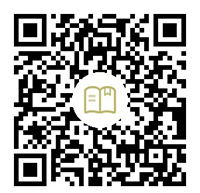# Tkinter 教程 布局管理

Tkinter 有三种布局方法，`pack``grid` 以及 `place` 方法。我们会对它们一一进行介绍。

## Tkinter pack 布局方法

`pack` 按照字面理解，就是打包的意思，它将刚创建的控件通过打包的方法来放置到窗口中。我们在 Tkinter 标签的学习章节中，第一次使用这种布局方法，并且列出了 pack 的所有选项。

### Tkinter pack 布局-相对位置

``````import tkinter as tk

app = tk.Tk()
app.geometry('300x200')

buttonW = tk.Button(app, text="West", width=15)
buttonW.pack(side='left')

buttonE = tk.Button(app, text="East", width=15)
buttonE.pack(side='right')

app.mainloop()
``````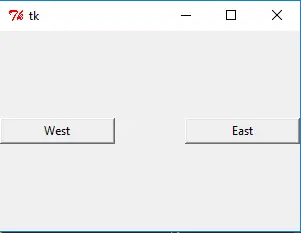``````buttonW.pack(side='left')
``````

side 有四个选项，topbottomleftright 。它会将控件放在窗口的 side 侧。就像所举的例子中，buttonW 放在窗口的左侧，因为 `side='left'`，而 buttonE 放在窗口的右侧，因为其 `side=right`

``````import tkinter as tk

app = tk.Tk()
app.geometry('300x200')

buttonW = tk.Button(app, text="West", width=15)
buttonW.pack(side='left')

buttonE1 = tk.Button(app, text="East 1", width=15)
buttonE1.pack(side='right')

buttonE2 = tk.Button(app, text="East 2", width=15)
buttonE2.pack(side='right')

app.mainloop()
``````

``````import tkinter as tk

app = tk.Tk()
app.geometry('300x200')

buttonW = tk.Button(app, text="West")

buttonE = tk.Button(app, text="East")

app.mainloop()
``````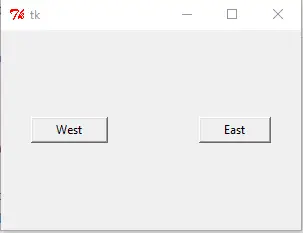### Tkinter pack 布局在 x,y 方向上的填充

``````import tkinter as tk

app = tk.Tk()
app.geometry('300x200')

buttonX = tk.Button(app, text="Fill X", bg="red", height=5)
buttonX.pack(fill='x')

buttonY = tk.Button(app, text="Fill Y", bg="green", width=10)
buttonY.pack(side='left', fill='y')

app.mainloop()
```````butonX.pack(fill='x')` 意味着控件 buttonX 的宽度会填充满整个窗口的宽度，同样的，如果 `fill='y'` 就将控件的高度延伸至填满整个窗口的高度，fill='both'就是在 X 和 Y 方向的结合，会自动在宽度和高度方向上填满整个窗口。

### Tkinter pack 布局 expand 选项-自动展开控件

``````import tkinter as tk
import calendar

app = tk.Tk()

buttonX = tk.Button(app, text="Label ", bg="blue", height=5)
buttonX.pack(fill='x')

listboxA = tk.Listbox(app, width=10)
listboxA.pack(fill='both', expand=1)

for i in range(1,13):
listboxA.insert(tk.END, calendar.month_name[i])

app.mainloop()
``````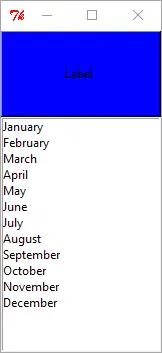`expand=True or 1` 时，列表框就会铺开列表中所有的元素，比如例子中，显示了从 January 到 December。

``````listboxA.pack(fill='both', expand=0)
``````### Tkinter grid 布局方法

Tkinter grid 是另外一种，也是最重要的一种窗口内控件布局的方法，假如要在三种布局方法里面只学习一种的话，那非 `grid` 方法莫属。

`grid` 经常用在用在对话框中，你可以按照网格的坐标位置来安放控件，这样能够得到稳定的控件相对位置。

``````import tkinter as tk

app = tk.Tk()

labelWidth = tk.Label(app,
text = "Width Ratio")

labelHeight = tk.Label(app,
text = "Height Ratio")

entryWidth = tk.Entry(app, width=20)
entryHeight = tk.Entry(app, width=20)

resultButton = tk.Button(app, text = 'Get Result')

logo = tk.PhotoImage(file='python.gif')
labelLogo = tk.Label(app, image=logo)

labelLogo.grid(row=0, column=2, columnspan=2, rowspan=2,

app.mainloop()
``````### Tkinter grid column 和 row 选项

``````labelWidth.grid(column=0, row=0, ipadx=5, pady=5, sticky=tk.W+tk.N)
``````

`labelWidth` 控件就放在坐标为 (0, 0) 的单元格中，坐标是以窗口的左上角为坐标系的原点。

## Tkinter grid sticky 选项

sticky 的作用是当所产生的单元格大小比控件本身要大的时候，如何进行扩展。

sticky 选项 意义
W 左对齐
E 右对齐
N 上对齐
S 下对齐

`sticky` 的默认选项是居中，也就是 `W+E+N+S`

### Tkinter columnspan 和 rowspan 选项

``````labelLogo.grid(row=0, column=2, columnspan=2, rowspan=2,
``````

## Tkinter place 方法

Tkinter place 是将控件安放在窗口中的固定或者相对的位置上。我们还是同样的采用刚才类似的思路，先列出例子和图形界面来，然后再来解释具体的选项。

``````import tkinter as tk

app = tk.Tk()
app.geometry('300x300')

labelA = tk.Label(app, text = "Label (0, 0)", fg="blue", bg="#FF0")
labelB = tk.Label(app, text = "Label (20, 20)", fg="green", bg="#300")
labelC = tk.Label(app, text = "Label (40, 50)", fg="black", bg="#f03")
labelD = tk.Label(app, text = "Label (0.5, 0.5)", fg="orange", bg="#0ff")

labelA.place(x=0, y=0)
labelB.place(x=20, y=20)
labelC.place(x=40, y=50)
labelD.place(relx=0.5, rely=0.5)

app.mainloop()
``````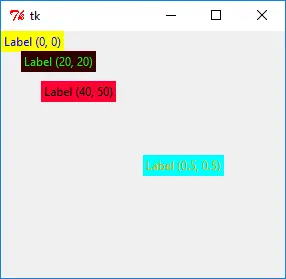### Tkinter place 绝对位置

``````labelA.place(x=0, y=0)
labelB.place(x=20, y=20)
``````

place 中 `x=``y=` 选项确定了控件的绝对位置，它们的单位是像素。比如 `lableB.place(x=20, y=20)` 的意思就是将控件放置在坐标为 (20, 20) 的位置上。

### Tkinter place 相对位置

``````labelD.place(relx=0.5, rely=0.5)
``````

`relx=1.0` 的位置是窗口的右边框，`rely=1.0` 的位置是窗口的下边框。

##  查看笔记